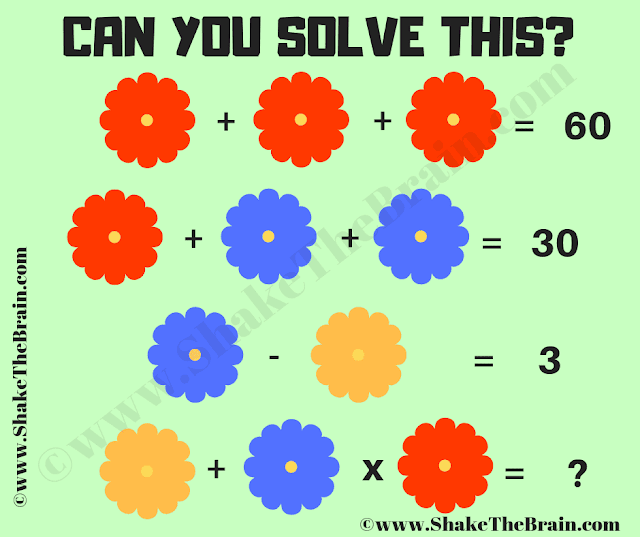Engage with an algebra problem: a math picture brain teaser. Challenge yourself and discover the answer to this captivating puzzle.

It is a simple math equation picture puzzle to test your brain. This is an easy math puzzle. However, if you are not clear with the PEMDAS or BODMAS concepts, you may get a different answer. Let us see if you can answer this math equation picture puzzle correctly and find the value of the missing number which replaces the question mark?Can you find the value of the missing number?

The answer to this "Math Equations Picture Puzzle", can be viewed by clicking the answer button.